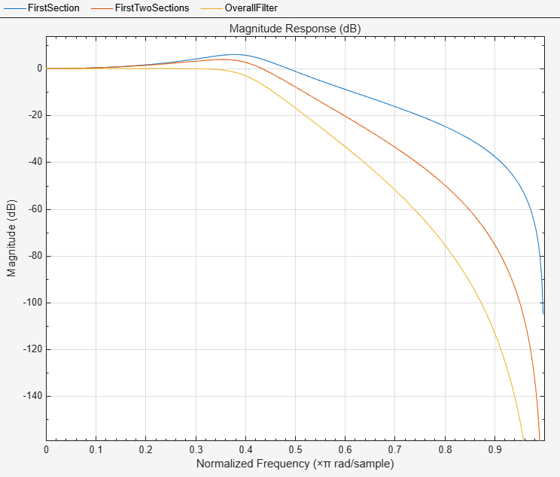cumsec

Cumulative second-order section of the biquadratic filter

Description

example

sect = cumsec(sysobj) returns a cell array, sect, which contains cumulative sections of the biquadratic filter System object™, sysobj. Each element in sect is a filter with the structure of the original filter. The first element is the first filter section of the biquadratic filter. The second element of sect is a filter that represents the combination of the first and second sections of the biquadratic filter. The third element of sect is a filter which combines sections 1, 2, and 3 of the biquadratic filter. This pattern continues until the final element of sect contains all the sections of the biquadratic filter and should be identical to the biquadratic filter.

sect = cumsec(sysobj,indices) returns the cumulative sections of the biquadratic filter object whose indices in the original filter are in the vector indices.

sect = cumsec(sysobj,indices,secondary) uses the secondary scaling points secondary in the sections to determine where the sections should be split when secondary is true. secondary is false by default. This option only applies for biquadratic filter objects with 'Direct form II' and 'Direct form I transposed' structures. For these structures, the secondary scaling points refer to the location between the recursive and the nonrecursive part, that is the 'middle' of the section.

cumsec(sysobj,...) plots the magnitude response of the cumulative sections using fvtool.

sect = cumsec(sysobj,'Arithmetic',arithType) returns the cumulative sections of the biquadratic filter object with the arithmetic specified in arithType.

Examples

collapse all

This example plots the relative responses of the sections of a sixth-order filter with three sections. Each curve adds one more section to form the filter response.

Lowpass = fdesign.lowpass('n,fc',6,.4); ButterLowpass = butter(Lowpass,'SystemObject',true);
CumSections = cumsec(ButterLowpass); hfvt = fvtool(CumSections{1},CumSections{2},CumSections{3});
legend(hfvt,'First Section','First Two Sections','Overall  Filter');Input Arguments

collapse all

Input filter, specified as one of the following System objects:

Filter indices. Use indices to specify the filter sections cumsec uses to compute the cumulative responses.

This option applies only when sysobj has the df2sos and df1tsos structures. For these second-order section structures, the secondary scaling points refer to the scaling locations between the recursive and the nonrecursive parts of the section (the "middle" of the section). Argument secondary accepts either true or false. By default, secondary is false.

Arithmetic used in the filter analysis, specified as 'double', 'single', or 'Fixed'. When the arithmetic input is not specified and the filter System object is unlocked, the analysis tool assumes a double-precision filter. When the arithmetic input is not specified and the System object is locked, the function performs the analysis based on the data type of the locked input.

The 'Fixed' value applies to filter System objects with fixed-point properties only.

When the 'Arithmetic' input argument is specified as 'Fixed' and the filter object has the data type of the coefficients set to 'Same word length as input', the arithmetic analysis depends on whether the System object is unlocked or locked.

• unlocked –– The analysis object function cannot determine the coefficients data type. The function assumes that the coefficients data type is signed, has a 16-bit word length, and is auto scaled. The function performs fixed-point analysis based on this assumption.

• locked –– When the input data type is 'double' or 'single', the analysis object function cannot determine the coefficients data type. The function assumes that the data type of the coefficients is signed, has a 16-bit word length, and is auto scaled. The function performs fixed-point analysis based on this assumption.

To check if the System object is locked or unlocked, use the isLocked function.

When the arithmetic input is specified as 'Fixed' and the filter object has the data type of the coefficients set to a custom numeric type, the object function performs fixed-point analysis based on the custom numeric data type.

Objects

Introduced in R2011a

DSP System Toolbox Documentation